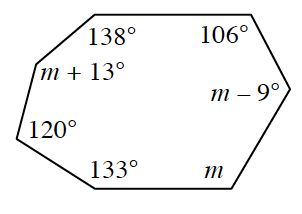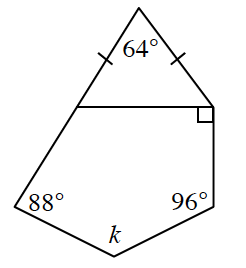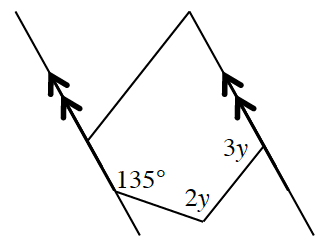### Home > INT2 > Chapter 8 > Lesson 8.4.3 > Problem8-106

8-106.

Use the angle relationships in each of the diagrams below to solve for the given variables. Show all work.

The sum of the interior angles in an $n$-gon can be found by calculating $\left(n-2\right)180^{\circ}$.

1.$m = 133^\circ$

1.Start by determining the measures of the base angles of the isosceles triangle.

1.The sum of the two unlabeled angles is $180^\circ$. Why?